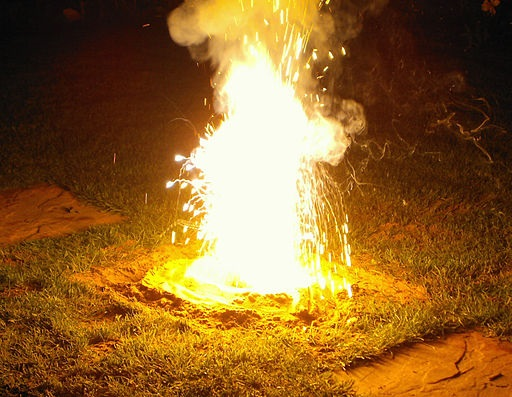Chemistry Edexcel GCSE:
Calculations 1b

For Double and Triple sciences

Grab a calculator and a copy of the periodic table

(This test has only 10 questions, but may take 15 - 20 mins).

Q1-2:  A student used the following reaction to try to produce 20.0 g of copper(II)chloride.

CuCO3 + 2HCl  CuCl2  + H2O  + CO2

[Relative atomic masses Cl = 35.5; Cu = 63.5]

1. The formula mass of copper(II)chloride and the number of moles of copper(II)chloride produced is …

 Formula Mass Moles produced A 99 4.95 B 99 0.202 C 134.5 6.73 D 134.5 0.149
2. What mass of copper(II)carbonate does the student need to react with excess hydrochloric acid to make the 20.0 g of copper(II)chloride? [Mr of CuCO3 = 123.5]
• A.   18.4 g
• B.   20.0 g
• C.   24.9 g
• D.   36.8 g
3. Which of the following statements about chemical reactions is true?

The total mass of the reactants is …..

• A.  .. equal to the total mass of the products in all chemical reactions
• B.   ..less than the total mass of the products in a precipitation reaction in a closed flask
• C.   ..is greater than the total mass of the products in a precipitation reaction in a closed flask
• D.  .. is greater than the total mass of the products in a reaction producing a gas in an open flask

4. In the thermite reaction aluminium powder reacts very exothermically with iron(III)oxide displacing molten iron. The equation for the reaction is:

2Al + Fe2O3  Al2O3 + 2Fe

What mass of molten iron is formed from 0.1moles of iron(III)oxide?
[Mr of Fe2O3 is 160; Ar of Fe is 56]• A.   2.8 g
• B.   5.6 g
• C.   11.2 g
• D.   22.4 g
5. 100cm3 of a sodium hydroxide solution was prepared by dissolving 0.2 moles of sodium hydroxide, NaOH, in water. [Mr of NaOH = 40]

What is the concentration of this solution in g dm-3?

• A.   4
• B.   8
• C.   40
• D.   80
6. How many CaCO3 particles are there in 2 moles of CaCO3?
• A.   1.20 x 1023
• B.   3.01 x 1023
• C.   1.02 x 1024
• D.   6.02 x 1024
7. What amount, in moles, is 3.0g of carbon and how many carbon atoms does it contain?

[Ar of carbon = 12]

 Number of moles of carbon Number of carbon atoms A 0.25 1.51 x 1023 B 0.25 2.41 x 1024 C 4.0 1.51 x 1023 D 4.0 2.41 x 1024

8. A mass of 3.00 g of magnesium was reacted with 0.200 moles of ethanoic acid in the following reaction:

Mg + 2CH3COOH  (CH3COO)2Mg + H2

Calculate the number of moles of magnesium used and determine the reagent in excess.

 Number of moles of magnesium Reagent in excess A 0.125 Ethanoic acid B 0.125 magnesium C 0.100 Ethanoic acid D 0.100 magnesium
9. An ore of titanium contains 36.8% iron, 31.6% titanium and 31.6% oxygen.

Determine the simplest whole number ratio for the moles of atoms.
[Ar values: Fe = 56; Ti = 48; O = 16]

 Fe Ti O A 1 1 1 B 1 1 2 C 1 1 3 D 4 3 1
10. Iron(III)hydroxide is a brown precipitate formed during the test for iron(III) ions.

FeCl3 (aq)  + 3NaOH (aq)  Fe(OH)3 (s)   +   3NaCl (aq)

What amount, in moles, of each reactant would be needed to produce 0.6 moles of iron(III)hydroxide?

 Moles of FeCl3 (aq) Moles of NaOH (aq) A 0.2 0.6 B 0.3 0.3 C 0.6 0.6 D 0.6 1.8